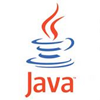# Data Structure: What is Stack ?

Connect withLearn what is Stack Data Structure? Stack is a simple data structure used for storing similar type. push() and pop() operation is largely used in Stack datastructure.

## 1. Key Points of Stack Data Structure

1. Stack is an ordered list, where insertion and deletion are permitted at one end only.
2. It is based on LIFO (Last-in First-out).
3. Stack is Linear List

## 2. Key operations in Stack data structure

The following are key operationss or methods in stack data structure. Basic operations of stack:

#### 2.1 Basic operations in Stack data structure

1. push(): method is used to push item on top of the stack.
2. pop(): method is used to return from top and delete.

#### 2.2 Supplementary operations in Stack

The supplimentary operations in stack are: `top()`, `peek()`, `size()` and `isEmpty()`

1. top()/peek(): it returns top element (last inserted element) from the stack without removing it.
2. size(): it returns number of element stored in the Stack.
3. isEmpty(): it returns boolean true/false whether element is available in stack or not

## 3. Different Implementation of Stack data structure

1. Simple Array based implementation: in the implementation size of array is fixed. A push operation will throw exception like fullStackException when you try to push in full arraylist.
2. Dynamic Array based implementation: this approach array are growable with predefined way.
3. LinkedList based implementation: in this approach , push method implemented by inseting element at the begining of the list. pop() method is implemented by deleting node from the beginning.

## 4. Application where Stack can be Used

There are a number of application based on stack which uses extensively in every languages. Few of application where stack being used as:

1. Implementing method chaining call
2. Page-visit history in web browser
3. Undo sequence in a text-editor
4. Matching tags in HTML/XML
5. Evaluation of postfix expression
6. infix to postfix conversion
7. Balancing symbol

## 5. Example of Stack in real life

There are number of real life example even for non-programmer one of them as

## 6. Analysis of Performance and Limitation

1. space complexity for pushing n element: `O(n)`
2. Time complexity of` push()`: `O(1)`
3. Time complexity of `pop()`: `O(1)`
4. Time complexity of `top()/peek()`: `O(1)`
5. Time complexity of `size()`: `O(1)`
6. Time complexity of `isEmpty()`: `O(1)`